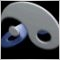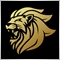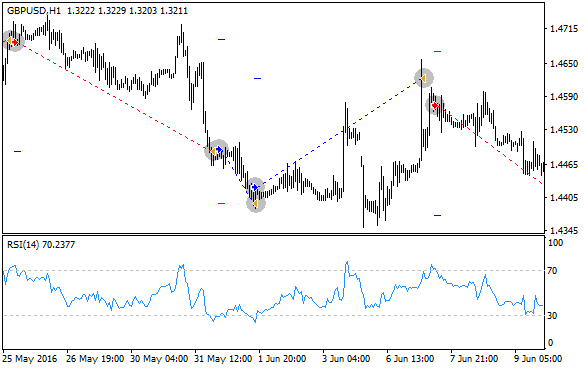# RSI EA code help341

Hi...

Need an indicator to trade RSI.

where if the value goes above 70 and retrace below 50 I enter short

wher if the value goes below 30 and retraces above 50 i enter long

need help with teh codeModerator
16562

Khurram Moied:

Hi...

Need an indicator to trade RSI.

where if the value goes above 70 and retrace below 50 I enter short

wher if the value goes below 30 and retraces above 50 i enter long

need help with teh code

Show the part of your code where you need help. We can't help you otherwise.18697

 Khurram Moied:  Need an indicator to trade RSI. Indicators can not trade, only EAs You have only three choices: Search for it, learn to code it, or pay (Freelance) someone to code it. We're not going to code it for you. We are willing to help you when you post your attempt (using SRC) and the nature of your problem.341

Keith Watford:
Show the part of your code where you need help. We can't help you otherwise.

Just need the guidance on how to program this.

can we do it with normal variables storing RSI values or need to build arraysModerator
10973

Khurram Moied:

Just need the guidance on how to program this.

can we do it with normal variables storing RSI values or need to build arrays

Both can work.

Normal for single symbol and array for multi symbol.341

Marco vd Heijden:
Both can work.

Normal for single symbol and array for multi symbol.
can you pls help with a sample code for thisModerator
10973

Well you can modify this one:

//+------------------------------------------------------------------+
//|                                               Moving Average.mq4 |
//|                   Copyright 2005-2014, MetaQuotes Software Corp. |
//|                                              http://www.mql4.com |
//+------------------------------------------------------------------+
#property copyright   "2005-2014, MetaQuotes Software Corp."
#property link        "http://www.mql4.com"
#property description "Moving Average sample expert advisor"

#define MAGICMA  20131111
//--- Inputs
input double Lots          =0.1;
input double MaximumRisk   =0.02;
input double DecreaseFactor=3;
input int    MovingPeriod  =12;
input int    MovingShift   =6;
//+------------------------------------------------------------------+
//| Calculate open positions                                         |
//+------------------------------------------------------------------+
int CalculateCurrentOrders(string symbol)
{
int buys=0,sells=0;
//---
for(int i=0;i<OrdersTotal();i++)
{
if(OrderSelect(i,SELECT_BY_POS,MODE_TRADES)==false) break;
if(OrderSymbol()==Symbol() && OrderMagicNumber()==MAGICMA)
{
if(OrderType()==OP_BUY)  buys++;
if(OrderType()==OP_SELL) sells++;
}
}
//--- return orders volume
if(buys>0) return(buys);
else       return(-sells);
}
//+------------------------------------------------------------------+
//| Calculate optimal lot size                                       |
//+------------------------------------------------------------------+
double LotsOptimized()
{
double lot=Lots;
int    orders=HistoryTotal();     // history orders total
int    losses=0;                  // number of losses orders without a break
//--- select lot size
lot=NormalizeDouble(AccountFreeMargin()*MaximumRisk/1000.0,1);
//--- calcuulate number of losses orders without a break
if(DecreaseFactor>0)
{
for(int i=orders-1;i>=0;i--)
{
if(OrderSelect(i,SELECT_BY_POS,MODE_HISTORY)==false)
{
Print("Error in history!");
break;
}
if(OrderSymbol()!=Symbol() || OrderType()>OP_SELL)
continue;
//---
if(OrderProfit()>0) break;
if(OrderProfit()<0) losses++;
}
if(losses>1)
lot=NormalizeDouble(lot-lot*losses/DecreaseFactor,1);
}
//--- return lot size
if(lot<0.1) lot=0.1;
return(lot);
}
//+------------------------------------------------------------------+
//| Check for open order conditions                                  |
//+------------------------------------------------------------------+
void CheckForOpen()
{
double ma;
int    res;
//--- go trading only for first tiks of new bar
if(Volume>1) return;
//--- get Moving Average
ma=iMA(NULL,0,MovingPeriod,MovingShift,MODE_SMA,PRICE_CLOSE,0);
//--- sell conditions
if(Open>ma && Close<ma)
{
res=OrderSend(Symbol(),OP_SELL,LotsOptimized(),Bid,3,0,0,"",MAGICMA,0,Red);
return;
}
//--- buy conditions
if(Open<ma && Close>ma)
{
res=OrderSend(Symbol(),OP_BUY,LotsOptimized(),Ask,3,0,0,"",MAGICMA,0,Blue);
return;
}
//---
}
//+------------------------------------------------------------------+
//| Check for close order conditions                                 |
//+------------------------------------------------------------------+
void CheckForClose()
{
double ma;
//--- go trading only for first tiks of new bar
if(Volume>1) return;
//--- get Moving Average
ma=iMA(NULL,0,MovingPeriod,MovingShift,MODE_SMA,PRICE_CLOSE,0);
//---
for(int i=0;i<OrdersTotal();i++)
{
if(OrderSelect(i,SELECT_BY_POS,MODE_TRADES)==false) break;
if(OrderMagicNumber()!=MAGICMA || OrderSymbol()!=Symbol()) continue;
//--- check order type
if(OrderType()==OP_BUY)
{
if(Open>ma && Close<ma)
{
if(!OrderClose(OrderTicket(),OrderLots(),Bid,3,White))
Print("OrderClose error ",GetLastError());
}
break;
}
if(OrderType()==OP_SELL)
{
if(Open<ma && Close>ma)
{
if(!OrderClose(OrderTicket(),OrderLots(),Ask,3,White))
Print("OrderClose error ",GetLastError());
}
break;
}
}
//---
}
//+------------------------------------------------------------------+
//| OnTick function                                                  |
//+------------------------------------------------------------------+
void OnTick()
{
//--- check for history and trading
if(Bars<100 || IsTradeAllowed()==false)
return;
//--- calculate open orders by current symbol
if(CalculateCurrentOrders(Symbol())==0) CheckForOpen();
else                                    CheckForClose();
//---
}
//+------------------------------------------------------------------+
or look in codebase. https://www.mql5.com/en/codeMQL5 Code Base
• www.mql5.com
MQL5 Source Code Library for MetaTrader 5341

Khurram Moied:
can you pls help with a sample code for this

I already have MA EA in MT4.

what i did ask for was a sample code to program RSI.

if RSI locks above 70 and then locks below 50 for a short trade signal

and similarly locks below 30 and then above 50 for a long signal.

I need help to program the above.

I know it is easy to store the value of indicator in a variable for the nth candle......but in this case it seems the RSI array needs to be built......or ??? need help here.

since we dont know how much will be gap of candles when rsi is above and goes below 50.......it seems to be a dynamic array.

Need help on how to program the above.Moderator
10973

And i told you go to CODE BASE you do not have to re invent the wheel.

Is it so hard to type RSI EA in the search box and press enter ?

LET ME HELP YOU.

https://www.mql5.com/en/code/16069

Here is one:

//+------------------------------------------------------------------+
//|                                                          RSI.mq4 |
//|                               Copyright © 2016, Хлыстов Владимир |
//|                                                cmillion@narod.ru |
//+------------------------------------------------------------------+
#property copyright "Copyright © 2016, Хлыстов Владимир"
#property link      "cmillion@narod.ru"
#property strict
#property description "советник по RSI"
#property description "sell при пересечение сверху вниз 70 и на buy снизу вверх 30"
#property description "стопы и тейки можно выстовить в настройках советника"
//--------------------------------------------------------------------
extern int     period_RSI           = 14,
stoploss             = 100,
takeprofit           = 200,
slippage             = 10,
buy_level            = 30,
sell_level           = 70,
Magic                = 777;
extern double  Lot                  = 0.1;
//--------------------------------------------------------------------
void OnTick()
{
for (int i=0; i<OrdersTotal(); i++)
if (OrderSelect(i,SELECT_BY_POS,MODE_TRADES))
if (OrderSymbol()==Symbol() && Magic==OrderMagicNumber()) return;
double RSI0  = iRSI(NULL,0,period_RSI,PRICE_OPEN,0);
double RSI1  = iRSI(NULL,0,period_RSI,PRICE_OPEN,1);
double SL=0,TP=0;
if (RSI0 > buy_level && RSI1 < buy_level)
{
if (takeprofit!=0) TP  = NormalizeDouble(Ask + takeprofit*Point,Digits);
if (stoploss!=0)   SL  = NormalizeDouble(Ask - stoploss*  Point,Digits);
if (OrderSend(Symbol(),OP_BUY, Lot,NormalizeDouble(Ask,Digits),slippage,SL,TP,NULL,Magic)==-1) Print(GetLastError());
}
if (RSI0 < sell_level && RSI1 > sell_level)
{
if (takeprofit!=0) TP = NormalizeDouble(Bid - takeprofit*Point,Digits);
if (stoploss!=0)   SL = NormalizeDouble(Bid + stoploss*  Point,Digits);
if (OrderSend(Symbol(),OP_SELL,Lot,NormalizeDouble(Bid,Digits),slippage,SL,TP,NULL,Magic)==-1) Print(GetLastError());
}
}
//--------------------------------------------------------------------Would that suit your needs?

It's readily available in code base with thousands of others.

Just SEARCH for it.

I won't re-invent the wheel for you i refuse.The simplest RSI-based EA
• votes: 22
• 2016.10.27
• Vladimir Khlystov
• www.mql5.com
Sells at downward crossing of 70, buys at the upward crossing of 30.341

Marco vd Heijden:

And i told you go to CODE BASE you do not have to re invent the wheel.

Is it so hard to type RSI EA in the search box and press enter ?

LET ME HELP YOU.

https://www.mql5.com/en/code/16069

Here is one:

//+------------------------------------------------------------------+
//|                                                          RSI.mq4 |
//|                               Copyright © 2016, Хлыстов Владимир |
//|                                                cmillion@narod.ru |
//+------------------------------------------------------------------+
#property copyright "Copyright © 2016, Хлыстов Владимир"
#property link      "cmillion@narod.ru"
#property strict
#property description "советник по RSI"
#property description "sell при пересечение сверху вниз 70 и на buy снизу вверх 30"
#property description "стопы и тейки можно выстовить в настройках советника"
//--------------------------------------------------------------------
extern int     period_RSI           = 14,
stoploss             = 100,
takeprofit           = 200,
slippage             = 10,
buy_level            = 30,
sell_level           = 70,
Magic                = 777;
extern double  Lot                  = 0.1;
//--------------------------------------------------------------------
void OnTick()
{
for (int i=0; i<OrdersTotal(); i++)
if (OrderSelect(i,SELECT_BY_POS,MODE_TRADES))
if (OrderSymbol()==Symbol() && Magic==OrderMagicNumber()) return;
double RSI0  = iRSI(NULL,0,period_RSI,PRICE_OPEN,0);
double RSI1  = iRSI(NULL,0,period_RSI,PRICE_OPEN,1);
double SL=0,TP=0;
if (RSI0 > buy_level && RSI1 < buy_level)
{
if (takeprofit!=0) TP  = NormalizeDouble(Ask + takeprofit*Point,Digits);
if (stoploss!=0)   SL  = NormalizeDouble(Ask - stoploss*  Point,Digits);
if (OrderSend(Symbol(),OP_BUY, Lot,NormalizeDouble(Ask,Digits),slippage,SL,TP,NULL,Magic)==-1) Print(GetLastError());
}
if (RSI0 < sell_level && RSI1 > sell_level)
{
if (takeprofit!=0) TP = NormalizeDouble(Bid - takeprofit*Point,Digits);
if (stoploss!=0)   SL = NormalizeDouble(Bid + stoploss*  Point,Digits);
if (OrderSend(Symbol(),OP_SELL,Lot,NormalizeDouble(Bid,Digits),slippage,SL,TP,NULL,Magic)==-1) Print(GetLastError());
}
}
//--------------------------------------------------------------------

Would that suit your needs?

It's readily available in code base with thousands of others.

Just SEARCH for it.

I won't re-invent the wheel for you i refuse.

Thanks for a sample to start with.

But this code reads two consecutive RSi values.

In this example RSI0 and RSI1 records only two recent RSI consecutive values only.

How can we store RSI values which are N shifts apart.

See example here the two RSI events >70 and <50 are about 10 shifts apart.

since everytime shifts are dynamically changing between two RSI events, how can we program this. Any idea or code avlbl?

Files:
Short_RSI.JPG 44 kbModerator
10973

YES

//+------------------------------------------------------------------+
//|                                                          RSI.mq4 |
//|                               Copyright © 2016, Хлыстов Владимир |
//|                                                cmillion@narod.ru |
//+------------------------------------------------------------------+
#property copyright "Copyright © 2016, Хлыстов Владимир"
#property link      "cmillion@narod.ru"
#property strict
#property description "советник по RSI"
#property description "sell при пересечение сверху вниз 70 и на buy снизу вверх 30"
#property description "стопы и тейки можно выстовить в настройках советника"
//--------------------------------------------------------------------
extern int     period_RSI           = 14,
stoploss             = 100,
takeprofit           = 200,
slippage             = 10,
buy_level            = 30,
sell_level           = 70,
Magic                = 777;
extern double  Lot                  = 0.1;
//--------------------------------------------------------------------
void OnTick()
{
for (int i=0; i<OrdersTotal(); i++)
if (OrderSelect(i,SELECT_BY_POS,MODE_TRADES))
if (OrderSymbol()==Symbol() && Magic==OrderMagicNumber()) return;
double RSI0  = iRSI(NULL,0,period_RSI,PRICE_OPEN,N);
double RSI1  = iRSI(NULL,0,period_RSI,PRICE_OPEN,N);
double SL=0,TP=0;
if (RSI0 > buy_level && RSI1 < buy_level)
{
if (takeprofit!=0) TP  = NormalizeDouble(Ask + takeprofit*Point,Digits);
if (stoploss!=0)   SL  = NormalizeDouble(Ask - stoploss*  Point,Digits);
if (OrderSend(Symbol(),OP_BUY, Lot,NormalizeDouble(Ask,Digits),slippage,SL,TP,NULL,Magic)==-1) Print(GetLastError());
}
if (RSI0 < sell_level && RSI1 > sell_level)
{
if (takeprofit!=0) TP = NormalizeDouble(Bid - takeprofit*Point,Digits);
if (stoploss!=0)   SL = NormalizeDouble(Bid + stoploss*  Point,Digits);
if (OrderSend(Symbol(),OP_SELL,Lot,NormalizeDouble(Bid,Digits),slippage,SL,TP,NULL,Magic)==-1) Print(GetLastError());
}
}
//--------------------------------------------------------------------
To add comments, please log in or register